# NCERT Solutions For Class 11 Physics chapter-7 System of Particles and Rotational Motion

## NCERT Solutions for Class 11 Physics

NCERT Solutions for class-11 Physics Chapter-7 System of Particles and Rotational Motion is prepared by our senior and renowned teachers of Physics Wallah primary focus while solving these questions of class-11 in NCERT textbook, also do read theory of this Chapter-7 System of Particles and Rotational Motion while going before solving the NCERT questions. You can download NCERT solution of all chapters from Physics Wallah in PDF.

### Chapter-7 System of Particles and Rotational Motion

Question 1. Give the location of the centre of mass of a (i) sphere, (ii) cylinder, (iii) ring, and (iv) cube, each of uniform mass density. Does the centre of mass of a body necessarily lie inside the body?

Solution :
In all the four cases, as the mass density is uniform, centreof mass is located at their respective geometrical centres.
No, it is not necessary that the centre of mass of a body should lie on the body. For example, in case of a circular ring, centre of mass is at the centre of the ring, where there is no mass.

Question 2. In the HCl molecule, the separation between the nuclei of the two atoms is about 1.27 Å (1 Å = 10–10 m). Find the approximate location of the CM of the molecule, given that a chlorine atom is about 35.5 times as massive as a hydrogen atom and nearly all the mass of an atom is concentrated in its nucleus.

Solution :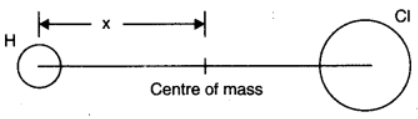Mass of H atom = m
Mass of Cl atom = 35.5m
Let the centre of mass of the system lie at a distance x from the Cl atom.
Distance of the centre of mass from the H atom = (1.27 – x)

Let us assume that the centre of mass of the given molecule lies at the origin. Therefore, we can have:
[ m(1.27 – x) + 35.5mx ] / (m + 35.5m)  =  0
m(1.27 – x) + 35.5mx =  0
1.27 - x = -35.5x
∴ x = -1.27 / (35.5 - 1)  =  -0.037 Å
Here, the negative sign indicates that the centre of mass lies at the left of the molecule. Hence, the centre of mass of the HCl molecule lies 0.037Å from the Cl atom.

Question 3. A child sits stationary at one end of a long trolley moving uniformly with a speed V on a smooth horizontal floor. If the child gets up and runs about on the trolley in any manner, what is the speed of the CM of the (trolley + child) system?

Solution :
The child is running arbitrarily on a trolley moving with velocity v. However, the running of the child will produce no effect on the velocity of the centre of mass of the trolley. This is because the force due to the boy’s motion is purely internal. Internal forces produce no effect on the motion of the bodies on which they act. Since no external force is involved in the boy–trolley system, the boy’s motion will produce no change in the velocity of the centre of mass of the trolley.

Question 4. Show that the area of the triangle contained between the vectors a and b is one half of the magnitude of a × b.

Solution :
Consider two vectors OK = vector |a| and OM = vector |b|, inclined at an angle θ, as shown in the following figure.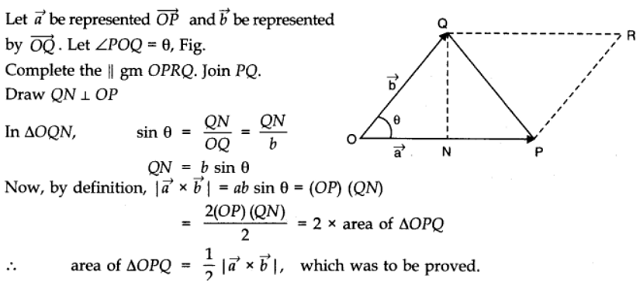Question 5. Show that a. (b × c) is equal in magnitude to the volume of the parallelepiped formed on the three vectors, a, b and c.

Solution :
A parallelepiped with origin O and sides a, b, and c is shown in the following figure.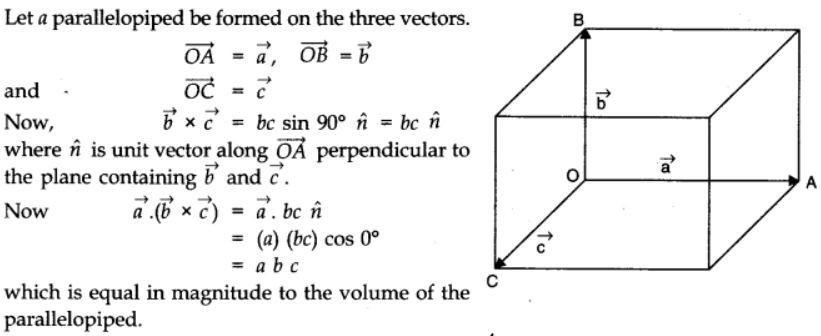Question 6. Find the components along the x, y, z axes of the angular momentum l of a particle, whose position vector is r with components x, y, z and momentum is p with components px, py and pz. Show that if the particle moves only in the x-yplane the angular momentum has only a z-component.

Solution :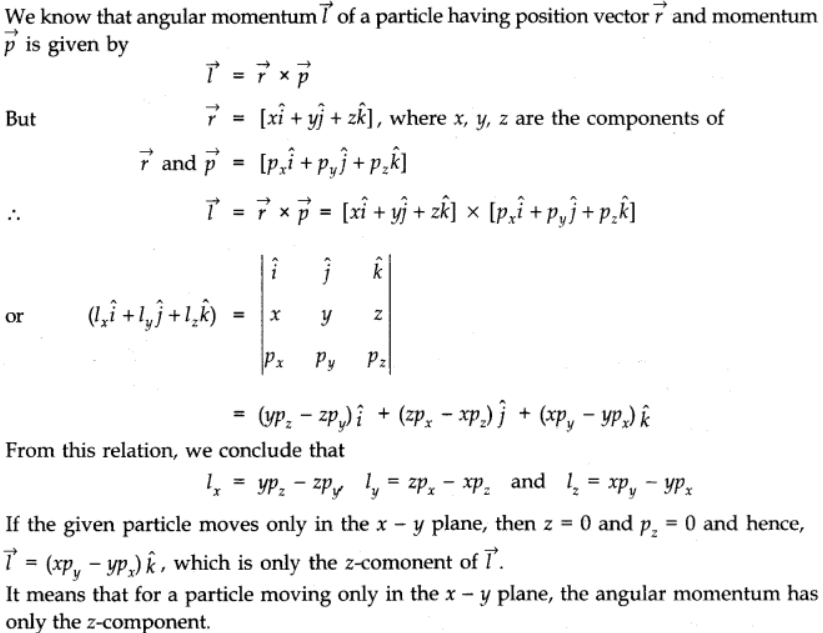Question 7. Two particles, each of mass m and speed v, travel in opposite directions along parallel lines separated by a distance d. Show that the vector angular momentum of the two particle system is the same whatever be the point about which the angular momentum is taken.

Solution :
Let at a certain instant two particles be at points P and Q, as shown in the following figure.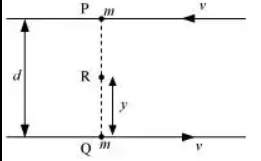Angular momentum of the system about point P: Lp = mv × 0 + mv × d  =  mvd   ...(i) Angular momentum of the system about point Q:
LQ = mv × d + mv × 0   =  mvd   ....(ii)
Consider a point R, which is at a distance y from point Q, i.e.,
QR = y
∴ PR = d – y
Angular momentum of the system about point R:
LR = mv × (d - y) + mv × y
mvd - mvy + mvy
= mvd  ....(iii) Comparing equations (i), (ii), and (iii), we get:
LP = LQ = LR    ...(iv)
We infer from equation (iv) that the angular momentum of a system does not depend on the point about which it is taken.

Question 8. A non-uniform bar of weight W is suspended at rest by two strings of negligible weight as shown in Fig.7.39. The angles made by the strings with the vertical are 36.9° and 53.1° respectively. The bar is 2 m long. Calculate the distance d of the centre of gravity of the bar from its left end.

\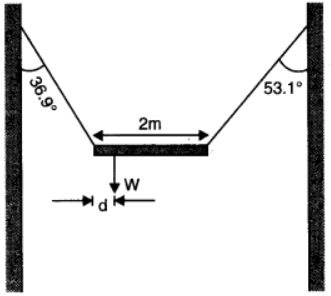Solution :
The free body diagram of the bar is shown in the following figure.

\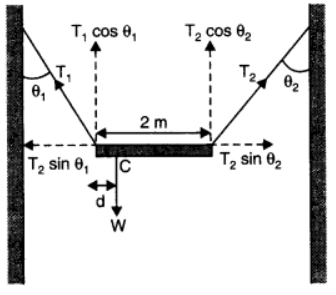Length of the bar, l = 2 m
T1 and T2 are the tensions produced in the left and right strings respectively.

At translational equilibrium, we have:
T1  Sin 36.90  = T2 Sin 53.10
T1 / T2 = 4 / 3
⇒ T1 = (4/3) T2
For rotational equilibrium, on taking the torque about the centre of gravity, we have:
T1  (Cos 36.9)  ×  d = T2  Cos 53.1  (2 - d)
T1 × 0.800 d = T2 × 0.600 (2 - d)
(4/3) × T2  ×  0.800d =  T2  (0.600  ×  2 -  0.600d)
1.067d  +  0.6d = 1.2
∴ d = 1.2 / 1.67
= 0.72 m
Hence, the C.G. (centre of gravity) of the given bar lies 0.72 m from its left end.

Question 9. A car weighs 1800 kg. The distance between its front and back axles is 1.8 m. Its centre of gravity is 1.05 m behind the front axle. Determine the force exerted by the level ground on each front wheel and each back wheel.

Solution :
Mass of the car, m = 1800 kg Distance between the front and back axles, d = 1.8 m
Distance between the C.G. (centre of gravity) and the back axle = 1.05 m
The various forces acting on the car are shown in the following figure.

\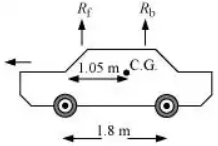Rf and Rbare the forces exerted by the level ground on the front and back wheels respectively. At translational equilibrium:
Rf + Rb = mg
= 1800 × 9.8
= 17640 N   ....(i) For rotational equilibrium, on taking the torque about the C.G., we have:
Rf(1.05) = Rb(1.8 - 1.05)
Rb / Rf  =  7 / 5
Rb = 1.4 Rf    ......(ii) Solving equations (i) and (ii), we get:
1.4Rf + Rf = 17640
Rf = 7350 N
∴ Rb = 17640 – 7350 = 10290 N
Therefore, the force exerted on each front wheel = 7350 / 2  =  3675 N, and
The force exerted on each back wheel = 10290 / 2  =  5145 N

Question 10. a. Find the moment of inertia of a sphere about a tangent to the sphere, given the moment of inertia of the sphere about any of its diameters to be 2MR2/5, where M is the mass of the sphere and R is the radius of the sphere.
b. Given the moment of inertia of a disc of mass M and radius R about any of its diameters to be MR2/4, find its moment of inertia about an axis normal to the disc and passing through a point on its edge.

Solution :
a. The moment of inertia (M.I.) of a sphere about its diameter = 2MR2/5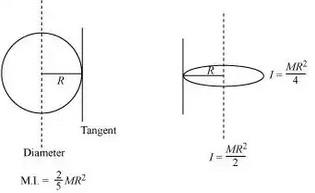According to the theorem of parallel axes, the moment of inertia of a body about any axis is equal to the sum of the moment of inertia of the body about a parallel axis passing through its centre of mass and the product of its mass and the square of the distance between the two parallel axes. The M.I. about a tangent of the sphere = 2MR2/5 + MR =  7MR2 / 5

b. The moment of inertia of a disc about its diameter = MR2 / 4
According to the theorem of perpendicular axis, the moment of inertia of a planar body (lamina) about an axis perpendicular to its plane is equal to the sum of its moments of inertia about two perpendicular axes concurrent with perpendicular axis and lying in the plane of the body.
The M.I. of the disc about its centre = MR2 / 4 + MR2 / 4  =  MR2 / 2
Applying the theorem of parallel axes:
The moment of inertia about an axis normal to the disc and passing through a point on its edge
= MR2 / 2 + MR2 = 3MR2 / 2

Question 11. Torques of equal magnitude are applied to a hollow cylinder and a solid sphere, both having the same mass and radius. The cylinder is free to rotate about its standard axis of symmetry, and the sphere is free to rotate about an axis passing through its centre. Which of the two will acquire a greater angular speed after a given time?

Solution :
Let m and r be the respective masses of the hollow cylinder and the solid sphere.
The moment of inertia of the hollow cylinder about its standard axis, I1 = mr2
The moment of inertia of the solid sphere about an axis passing through its centre, I2 = (2/5)mr2
We have the relation:
τ = Iα
Where,
α = Angular acceleration
τ = Torque
I = Moment of inertia
For the hollow cylinder, τ1 = I1α1
For the solid sphere, τn = Inαn
As an equal torque is applied to both the bodies, τ1 = τ2
∴ α2 / α1  =  I1  /  I2  =  mr2  /  (2/5)mr2
α2 > α1     ...(i)
Now, using the relation:
ω = ω0 + αt
Where,
ω0 = Initial angular velocity
t = Time of rotation
ω = Final angular velocity
For equal ω0 and t, we have:
ω ∝ α … (ii) From equations (i) and (ii), we can write:
ω2 > ω1
Hence, the angular velocity of the solid sphere will be greater than that of the hollow cylinder.

Question 12. A solid cylinder of mass 20 kg rotates about its axis with angular speed 100 rad s–1. The radius of the cylinder is 0.25 m. What is the kinetic energy associated with the rotation of the cylinder? What is the magnitude of angular momentum of the cylinder about its axis?

Solution :
Mass of the cylinder, m = 20 kg
Angular speed, ω = 100 rad s–1
Radius of the cylinder, r = 0.25 m
The moment of inertia of the solid cylinder:
I = mr2 / 2
= (1/2) × 20 × (0.25)2
= 0.625 kg m2
∴ Kinetic energy = (1/2) I ω2
= (1/2) × 6.25 × (100)2 = 3125 J
∴Angular momentum, L = Iω
= 6.25 × 100
= 62.5 Js

Question 13. a. A child stands at the centre of a turntable with his two arms outstretched. The turntable is set rotating with an angular speed of 40 rev/min. How much is the angular speed of the child if he folds his hands back and thereby reduces his moment of inertia to 2/5 times the initial value? Assume that the turntable rotates without friction.
b. Show that the child’s new kinetic energy of rotation is more than the initial kinetic energy of rotation. How do you account for this increase in kinetic energy?

Solution :
a. 100 rev/min Initial angular velocity, ω1= 40 rev/min
Final angular velocity = ω2
The moment of inertia of the boy with stretched hands = I1
The moment of inertia of the boy with folded hands = I2
The two moments of inertia are related as:
I2 = (2/5) I1
Since no external force acts on the boy, the angular momentum L is a constant.
Hence, for the two situations, we can write:
I2ω2  =  I1 ω1
ω2 = (I1/I2) ω1
= [ I1 / (2/5)I1 ] × 40  =  (5/2) × 40  =  100 rev/min

b.  Final K.E. = 2.5 Initial K.E.
Final kinetic rotation, EF = (1/2) I2 ω22
Initial kinetic rotation, EI =  (1/2) I1 ω12
EF / EI = (1/2) I2 ω22 / (1/2) I1 ω12
= (2/5) I1 (100)2 / I1 (40)2
= 2.5
∴ EF = 2.5 E1
The increase in the rotational kinetic energy is attributed to the internal energy of the boy.

Question 14. A rope of negligible mass is wound round a hollow cylinder of mass 3 kg and radius 40 cm. What is the angular acceleration of the cylinder if the rope is pulled with a force of 30 N? What is the linear acceleration of the rope? Assume that there is no slipping.

Solution :
Mass of the hollow cylinder, m = 3 kg
Radius of the hollow cylinder, r = 40 cm = 0.4 m
Applied force, F = 30 N
The moment of inertia of the hollow cylinder about its geometric axis:
I = mr2
= 3 × (0.4)2 = 0.48 kg m2
Torque, τ = F × r  =  30 × 0.4  = 12 Nm
For angular acceleration α, torque is also given by the relation:
τ = Iα
α = τ / I  =  12 / 0.48  = 25 rad s-2
Linear acceleration = τα = 0.4 × 25 = 10 m s–2

Question 15. To maintain a rotor at a uniform angular speed of 200 rad s–1, an engine needs to transmit a torque of 180 Nm. What is the power required by the engine?
(Note: uniform angular velocity in the absence of friction implies zero torque. In practice, applied torque is needed to counter frictional torque). Assume that the engine is 100 % efficient.

Solution :
Angular speed of the rotor, ω = 200 rad/s
Torque required, τ = 180 Nm
The power of the rotor (P) is related to torque and angular speed by the relation:
P = τω
= 180 × 200 = 36 × 103
= 36 kW
Hence, the power required by the engine is 36 kW.

Question 16. From a uniform disk of radius R, a circular hole of radius R/2 is cut out. The centre of the hole is at R/2 from the centre of the original disc. Locate the centre of gravity of the resulting flat body.

Solution :
R/6; from the original centre of the body and opposite to the centre of the cut portion. Mass per unit area of the original disc = σ
Radius of the original disc = R
Mass of the original disc, M = πR2σ
The disc with the cut portion is shown in the following figure: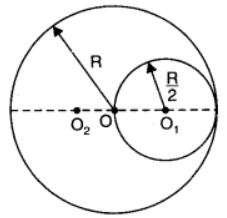Radius of the smaller disc = R/2 Mass of the smaller disc, M = π (R/2)2σ = π R2σ / 4  =  M / 4
Let O and O′ be the respective centres of the original disc and the disc cut off from the original. As per the definition of the centre of mass, the centre of mass of the original disc is supposed to be concentrated at O, while that of the smaller disc is supposed to be concentrated at O′.
It is given that:
OO′= R/2
After the smaller disc has been cut from the original, the remaining portion is considered to be a system of two masses. The two masses are:
M (concentrated at O), and –M′ (=M/4) concentrated at O′
(The negative sign indicates that this portion has been removed from the original disc.)
Let x be the distance through which the centre of mass of the remaining portion shifts from point O.
The relation between the centres of masses of two masses is given as:
x = (m1 r1 + m2 r2) /  (m1 + m2)
For the given system, we can write:
x = [ M × 0 - M' × (R/2) ] /  ( M + (-M') )  =  -R / 6
(The negative sign indicates that the centre of mass gets shifted toward the left of point O.)

Question 17. A metre stick is balanced on a knife edge at its centre. When two coins, each of mass 5 g are put one on top of the other at the 12.0 cm mark, the stick is found to be balanced at 45.0 cm. What is the mass of the metre stick?

Solution :
Let W and W′ be the respective weights of the metre stick and the coin.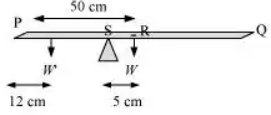The mass of the metre stick is concentrated at its mid-point, i.e., at the 50 cm mark. Mass of the meter stick = m
Mass of each coin, m = 5 g
When the coins are placed 12 cm away from the end P, the centre of mass gets shifted by 5 cm from point R toward the end P. The centre of mass is located at a distance of 45 cm from point P.
The net torque will be conserved for rotational equilibrium about point R.
10 × g(45 - 12) - m'g(50 - 45) = 0
∴ m' = 66 g
Hence, the mass of the metre stick is 66 g.

Question 18. A solid sphere rolls down two different inclined planes of the same heights but different angles of inclination.

(a) Will it reach the bottom with the same speed in each case?

(b) Will it take longer to roll down one plane than the other?

(c) If so, which one and why?

Solution :
(a) Mass of the sphere = m
Height of the plane = h
Velocity of the sphere at the bottom of the plane = v
At the top of the plane, the total energy of the sphere = Potential energy = mgh
At the bottom of the plane, the sphere has both translational and rotational kinetic energies.
Hence, total energy = (1/2)mv2 + (1/2) I ω2
Using the law of conservation of energy, we can write:
(1/2)mv2 + (1/2) I ω2 = mgh
For a solid sphere, the moment of inertia about its centre, I = (2/5)mr2
Hence, equation (i) becomes:
(1/2)mr2 + (1/2) [(2/5)mr22  =  mgh
(1/2)v2 + (1/5)r2ω2  =  gh
But we have the relation, v = rω
∴ (1/2)v2 + (1/5)v2  =  gh
v2(7/10) = gh
v =   √(10/7)gh
Hence, the velocity of the sphere at the bottom depends only on height (h) and acceleration due to gravity (g). Both these values are constants. Therefore, the velocity at the bottom remains the same from whichever inclined plane the sphere is rolled.

(b) Consider two inclined planes with inclinations θ1 and θ2, related as:
θ1 < θ2
The acceleration produced in the sphere when it rolls down the plane inclined at θ1 is:
g sin θ1
The various forces acting on the sphere are shown in the following figure.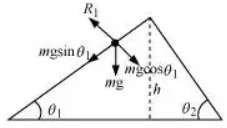R1 is the normal reaction to the sphere. Similarly, the acceleration produced in the sphere when it rolls down the plane inclined at θ2 is:
g sin θ2

The various forces acting on the sphere

R2 is the normal reaction to the sphere.
θ2 > θ1; sin θ2 > sin θ1 ... (i)
∴ a2 > a1 … (ii)
Initial velocity, u = 0
Final velocity, v = Constant
Using the first equation of motion, we can obtain the time of roll as:
v = u + at
∴ t ∝ (1/α)
For inclination θ1 : t1 ∝ (1/α1)
For inclination θ2 : t2 ∝ (1/α2)

From above equations, we get:
t2 < t1
Hence, the sphere will take a longer time to reach the bottom of the inclined plane having the smaller inclination.

Question 19. A hoop of radius 2 m weighs 100 kg. It rolls along a horizontal floor so that its centre of mass has a speed of 20 cm/s. How much work has to be done to stop it?

Solution :
Radius of the hoop, r = 2 m
Mass of the hoop, m = 100 kg
Velocity of the hoop, v = 20 cm/s = 0.2 m/s
Total energy of the hoop = Translational K.E. + Rotational K.E.
ET = (1/2)mv2 + (1/2) I ω2
Moment of inertia of the hoop about its centre, I = mr2
ET = (1/2)mv2 + (1/2) (mr22
But we have the relation, v = rω
∴ ET = (1/2)mv2 + (1/2)mr2ω2
= (1/2)mv2 + (1/2)mv2 = mv2
The work required to be done for stopping the hoop is equal to the total energy of the hoop.
∴ Required work to be done, W = mv2 = 100 × (0.2)2 = 4 J.

Question 20. The oxygen molecule has a mass of 5.30 × 10–26 kg and a moment of inertia of 1.94×10–46 kg m2 about an axis through its centre perpendicular to the lines joining the two atoms. Suppose the mean speed of such a molecule in a gas is 500 m/s and that its kinetic energy of rotation is two thirds of its kinetic energy of translation. Find the average angular velocity of the molecule.

Solution :
Mass of an oxygen molecule, m = 5.30 × 10–26 kg Moment of inertia, I = 1.94 × 10–46 kg m2
Velocity of the oxygen molecule, v = 500 m/s
The separation between the two atoms of the oxygen molecule = 2r
Mass of each oxygen atom = m/2
Hence, moment of inertia I, is calculated as:
(m/2)r2 + (m/2)r2 = mr2
r = ( I / m)1/2
(1.94 × 10-46 / 5.36 × 10-26 )1/2  =  0.60 × 10-10 m
It is given that:
KErot = (2/3)KEtrans
(1/2) I ω2 = (2/3) × (1/2) × mv2
mr2ω2 = (2/3)mv2
ω = (2/3)1/2 (v/r)
= (2/3)1/2 (500 / 0.6 × 10-10) = 6.80 × 1012 rad/s.

Question 21. A solid cylinder rolls up an inclined plane of angle of inclination 30°. At the bottom of the inclined plane the centre of mass of the cylinder has a speed of 5 m/s.
a. How far will the cylinder go up the plane?

Solution :

Given,

initial velocity of the solid cylinder, v = 5 m/s
Angle of inclination, θ = 30°
Assuming that the cylinder goes up to a height of h. We get :
( ½ ) mv2 + ( ½ ) l ω2mgh

( ½ ) mv2 + ( ½ ) ( ½ mr2) ω2mgh
3/4 mv2mgh (since v = rω)
h = 3v2 / 4g = (3 × 52) / 4 × 9.8 = 75/39.2 = 1.913 m

let d be the distance the cylinder covers up the plane, this means :
sin θ = h/d
d = h/sin θ  = 1.913/sin 30° = 3.826m
Now, the time required to return back: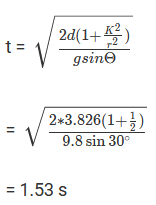Question 22. As shown in Fig.7.40, the two sides of a step ladder BA and CA are 1.6 m long and hinged at A. A rope DE, 0.5 m is tied half way up. A weight 40 kg is suspended from a point F, 1.2 m from B along the ladder BA. Assuming the floor to be frictionless and neglecting the weight of the ladder, find the tension in the rope and forces exerted by the floor on the ladder. (Take g = 9.8 m/s2) (Hint: Consider the equilibrium of each side of the ladder separately.)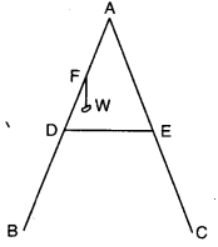Solution :
The given situation can be shown as: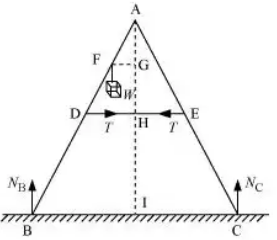NB = Force exerted on the ladder by the floor point B
NC = Force exerted on the ladder by the floor point C
T = Tension in the rope
BA = CA = 1.6 m
DE = 0. 5 m
BF = 1.2 m
Mass of the weight, m = 40 kg
Draw a perpendicular from A on the floor BC. This intersects DE at mid-point H.
ΔABI and ΔAIC are similar
∴BI = IC
Hence, I is the mid-point of BC.
DE || BC
BC = 2 × DE = 1 m
AF = BA – BF = 0.4 m … (i) D is the mid-point of AB.
Hence, we can write:
AD = (1/2) × BA  =  0.8 m   ...(ii) Using equations (i) and (ii), we get:
FE = 0.4 m
Hence, F is the mid-point of AD.
FG||DH and F is the mid-point of AD. Hence, G will also be the mid-point of AH.
∴ FG / DH  = AF / AD
FG / DH  =  0.4 / 0.8  =  1 / 2
FG = (1/2) DH
= (1/2) × 0.25  =  0.125 m

= (0.82 - 0.252)1/2  =  0.76 m

For translational equilibrium of the ladder, the upward force should be equal to the downward force.
Nc + NB = mg = 392 … (iii) For rotational equilibrium of the ladder, the net moment about A is:
-NB × BI + mg × FG + NC × CI + T × AG - T × AG  =  0
-NB × 0.5 + 40 × 9.8 × 0.125 + NC × 0.5  =  0
(NC - NB) × 0.5 = 49
NC - NB = 98   .....(iv) Adding equations (iii) and (iv), we get:
NC = 245 N
NB = 147 N
For rotational equilibrium of the side AB, consider the moment about A.
-NB × BI + mg × FG + T × AG  =  0
-245 × 0.5 + 40 X 9.8 × 0.125 + T × 0.76  =  0
∴ T = 96.7 N.

Question 23. A man stands on a rotating platform, with his arms stretched horizontally holding a 5 kg weight in each hand. The angular speed of the platform is 30 revolutions per minute. The man then brings his arms close to his body with the distance of each weight from the axis changing from 90cm to 20cm. The moment of inertia of the man together with the platform may be taken to be constant and equal to 7.6 kg m2.
(a) What is his new angular speed? (Neglect friction.)
(b) Is kinetic energy conserved in the process? If not, from where does the change come about?

Solution :
(a) Moment of inertia of the man-platform system = 7.6 kg m2
Moment of inertia when the man stretches his hands to a distance of 90 cm:
2 × m r2
= 2 × 5 × (0.9)2
= 8.1 kg m2
Initial moment of inertia of the system, Ii = 7.6 + 8.1 = 15.7 kg m2
Angular speed, ωi = 300 rev/min
Angular momentum, Li = Iiωi  =  15.7 × 30   ....(i)
Moment of inertia when the man folds his hands to a distance of 20 cm:
2 × mr2
= 2 × 5 (0.2)2 = 0.4 kg m2
Final moment of inertia, If = 7.6 + 0.4 = 8 kg m2
Final angular speed = ωf
Final angular momentum, Lf = Ifωf = 0.79 ωf ...... (ii)
From the conservation of angular momentum, we have:
Iiωi  =  Ifωf
∴ ωf = 15.7 × 30  / 8  =  58.88 rev/min

(b) Kinetic energy is not conserved in the given process. In fact, with the decrease in the moment of inertia, kinetic energy increases. The additional kinetic energy comes from the work done by the man to fold his hands toward himself.

Question 24. A bullet of mass 10 g and speed 500 m/s is fired into a door and gets embedded exactly at the centre of the door. The door is 1.0 m wide and weighs 12 kg. It is hinged at one end and rotates about a vertical axis practically without friction. Find the angular speed of the door just after the bullet embeds into it.
(Hint: The moment of inertia of the door about the vertical axis at one end is ML2/3.)

Solution :
Mass of the bullet, m = 10 g = 10 × 10–3 kg
Velocity of the bullet, v = 500 m/s
Thickness of the door, L = 1 m
Radius of the door, r = m / 2
Mass of the door, M = 12 kg
Angular momentum imparted by the bullet on the door:
α = mvr
= (10 × 10-3 ) × (500) × (1/2)  =  2.5 kg m2 s-1    ...(i)
Moment of inertia of the door:
I = ML2 / 3
= (1/3) × 12 × 12 = 4 kgm2
But α = Iω
∴ ω = α / I
= 2.5 / 4

Question 25. Two discs of moments of inertia I1 and I2 about their respective axes (normal to the disc and passing through the centre), and rotating with angular speeds ω1 and ω2 are brought into contact face to face with their axes of rotation coincident.

a. What is the angular speed of the two-disc system?

b. Show that the kinetic energy of the combined system is less than the sum of the initial kinetic energies of the two discs. How do you account for this loss in energy? Take ω1 ≠ ω2.

Solution :
a. Moment of inertia of disc I = I1 Angular speed of disc I = ω1
Moment of inertia of disc I = I2
Angular speed of disc I = ω2
Angular momentum of disc I, L1 = I1ω1
Angular momentum of disc II, L2 = I2ω2
Total initial angular momentum Li = I1ω1 + I2ω2
When the two discs are joined together, their moments of inertia get added up.
Moment of inertia of the system of two discs, I = I1 + I2
Let ω be the angular speed of the system.
Total final angular momentum, LT = (I1 + I2) ω
Using the law of conservation of angular momentum, we have:
Li = LT
I1ω1 + I2ω2 = (I1 + I2
∴ ω = (I1ω1 + I2ω2) / (I1 + I2)

b. Kinetic energy of disc I, E1 = (1/2) I1ω12
Kinetic energy of disc II, E1 = (1/2) I2ω22
Total initial kinetic energy, Ei = (1/2) ( I1ω12 + I2ω22)
When the discs are joined, their moments of inertia get added up.
Moment of inertia of the system, I = I1 + I2
Angular speed of the system = ω
Final kinetic energy Ef:  =  (1/2) ( I1 + I2) ω2
=  (1/2) ( I1 + I2) [ (I1ω1 + I2ω2) / (I1 + I2) ]2
= (1/2) (I1ω1 + I2ω2)2 / (I1 + I2)
∴ Ei - Ef
= (1/2) ( I1ω12 + I2ω22) - (1/2) (I1ω1 + I2ω2)2 / (I1 + I2)
Solving the equation, we get
= I1 I21 - ω2)2 / 2(I1 + I2)
All the quantities on RHS are positive
∴ Ei - Ef > 0
Ei > Ef
The loss of KE can be attributed to the frictional force that comes into play when the two discs come in contact with each other.

Question 26. a. Prove the theorem of perpendicular axes.
(Hint: Square of the distance of a point (x, y) in the x–y plane from an axis through the origin perpendicular to the plane is x2 + y2).
b. Prove the theorem of parallel axes.
(Hint: If the centre of mass is chosen to be the origin ∑ miri = 0).

Solution :
a. The theorem of perpendicular axes states that the moment of inertia of a planar body (lamina) about an axis perpendicular to its plane is equal to the sum of its moments of inertia about two perpendicular axes concurrent with perpendicular axis and lying in the plane of the body.
A physical body with centre O and a point mass m,in the x–y plane at (x, y) is shown in the following figure.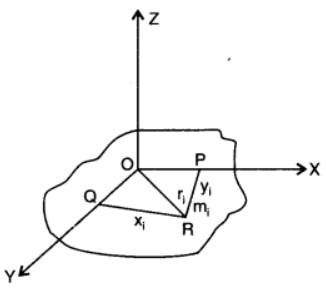Moment of inertia about x-axis, Ix = mx2 Moment of inertia about y-axis, Iy = my2
Moment of inertia about z-axis, Iz = m(x2 + y2)1/2

Ix + Iy = mx2 + my2
= m(x2 + y2)
= m [(x2 + y2)1/2]1/2
Ix + Iy = Iz
Hence, the theorem is proved.

b. The theorem of parallel axes states that the moment of inertia of a body about any axis is equal to the sum of the moment of inertia of the body about a parallel axis passing through its centre of mass and the product of its mass and the square of the distance between the two parallel axes.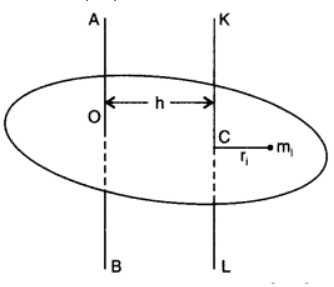Suppose a rigid body is made up of n particles, having masses m1, m2, m3, … , mn, at perpendicular distances r1, r2, r3, … , rn respectively from the centre of mass O of the rigid body.
The moment of inertia about axis RS passing through the point O:

Question 27. Prove the result that the velocity v of translation of a rolling body (like a ring, disc, cylinder or sphere) at the bottom of an inclined plane of a height h is given by v2 = 2gh / [1 + (k2/R2) ]
Using dynamical consideration (i.e. by consideration of forces and torques). Note k is the radius of gyration of the body about its symmetry axis, and R is the radius of the body. The body starts from rest at the top of the plane.

Solution :
A body rolling on an inclined plane of height h,is shown in the following figure: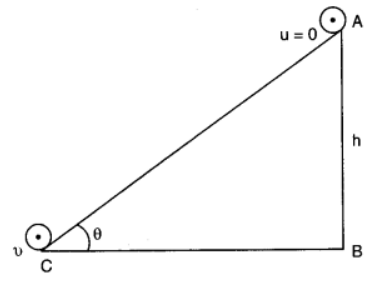m = Mass of the body
R = Radius of the body
K = Radius of gyration of the body
v = Translational velocity of the body
h =Height of the inclined plane
g = Acceleration due to gravity
Total energy at the top of the plane, E­1= mgh
Total energy at the bottom of the plane, Eb = KErot + KEtrans
= (1/2) I ω2 + (1/2) mv2
But I = mk2 and ω = v / R
∴ Eb = (1/2)(mk2)(v2/R2) + (1/2)mv2
= (1/2)mv2 (1 + k2 / R2)
From the law of conservation of energy, we have:
ET = Eb
mgh = (1/2)mv2 (1 + k2 / R2)
∴ v = 2gh / (1 + k2 / R2)
Hence, the given result is proved.

Question 28. A disc rotating about its axis with angular speed ωois placed lightly (without any translational push) on a perfectly frictionless table. The radius of the disc is R. What are the linear velocities of the points A, B and C on the disc shown in Fig. 7.41? Will the disc roll in the direction indicated?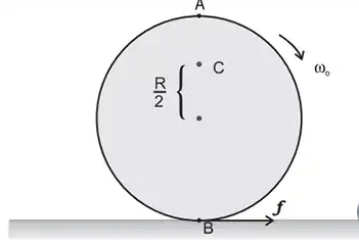vA = Rωo; vB = Rω0, vc = (R/2)ω0 The disc will not roll
Angular speed of the disc = ωo
Radius of the disc = R
Using the relation for linear velocity, v = ωoR
For point A:
vA = Rωo; in the direction tangential to the right
For point B:
vB = Rωo; in the direction tangential to the left
For point C:
vc = (R/2)ωo in the direction same as that of vA
The directions of motion of points A, B, and C on the disc are shown in the following figure .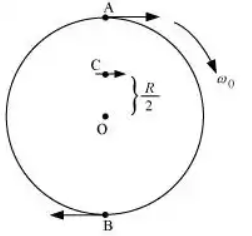Since the disc is placed on a frictionless table, it will not roll. This is because the presence of friction is essential for the rolling of a body.

Question 29. Explain why friction is necessary to make the disc in Fig. 7.41 roll in the direction indicated.

a. Give the direction of frictional force at B, and the sense of frictional torque, before perfect rolling begins.
b. What is the force of friction after perfect rolling begins?

A torque is required to roll the given disc. As per the definition of torque, the rotating force should be tangential to the disc. Since the frictional force at point B is along the tangential force at point A, a frictional force is required for making the disc roll.

a. Force of friction acts opposite to the direction of velocity at point B. The direction of linear velocity at point B is tangentially leftward. Hence, frictional force will act tangentially rightward. The sense of frictional torque before the start of perfect rolling is perpendicular to the plane of the disc in the outward direction.

b. Since frictional force acts opposite to the direction of velocity at point B, perfect rolling will begin when the velocity at that point becomes equal to zero. This will make the frictional force acting on the disc zero.

Question 30.A solid disc and a ring, both of radius 10 cm are placed on a horizontal table simultaneously, with initial angular speed equal to 10 π rad s-1. Which of the two will start to roll earlier? The co-efficient of kinetic friction is μk = 0.2.

Solution :
Radii of the ring and the disc, r = 10 cm = 0.1 m
Initial angular speed, ω0 =10 π rad s–1
Coefficient of kinetic friction, μk = 0.2
Initial velocity of both the objects, u = 0
Motion of the two objects is caused by frictional force. As per Newton’s second law of motion, we have frictional force, f = ma
μkmg= ma
Where,
a = Acceleration produced in the objects
m = Mass
∴ a = μkg … (i) As per the first equation of motion, the final velocity of the objects can be obtained as:
v = u + at
= 0 + μkgt
= μkgt … (ii) The torque applied by the frictional force will act in perpendicularly outward direction and cause reduction in the initial angular speed.
Torque, τ= –Iα
α = Angular acceleration
μkmgr = –Iα
∴ α = -μkmgr / I     .....(iii) Using the first equation of rotational motion to obtain the final angular speed:
ω = ω0 + αt
= ω0 + (-μkmgr / I)t    ....(iv)
Rolling starts when linear velocity, v = rω
∴ v = r (ω0 - μkmgrt / I)    ...(v)
Equating equations (ii) and (v), we get:
μkgt = r (ω0 - μkmgrt / I)
= rω0 - μkmgr2t / I    ....(vi)
For the ring:
I = mr2
∴ μkgt = rω0 - μkmgr2t / mr2
= rω0 - μkgt
2μkgt = rω0
∴ t = rω0 / 2μkg
= 0.1 × 10 × 3.14 / 2 × 0.2 × 9.8  =  0.80 s    ....(vii)
For the disc: I = (1/2)mr2
∴ μkgt = rω0 - μkmgr2t / (1/2)mr2
= rω0 - 2μkgt
3μkgt = rω0
∴ t = rω0 / 3μkg
= 0.1 × 10 × 3.14 / 3 × 0.2 × 9.8  =  0.53 s   .....(viii)
Since td > tr, the disc will start rolling before the ring.

Question 31. A cylinder of mass 10 kg and radius 15 cm is rolling perfectly on a plane of inclination 30°. The coefficient of static friction µs = 0.25.
a. How much is the force of friction acting on the cylinder?
b. What is the work done against friction during rolling?
c. If the inclination θ of the plane is increased, at what value of θ does the cylinder begin to skid, and not roll perfectly?

Solution :
Mass of the cylinder, m = 10 kg
Radius of the cylinder, r = 15 cm = 0.15 m
Co-efficient of kinetic friction, µk = 0.25
Angle of inclination, θ = 30°
Moment of inertia of a solid cylinder about its geometric axis, I = (1/2)mr2
The various forces acting on the cylinder are shown in the following figure: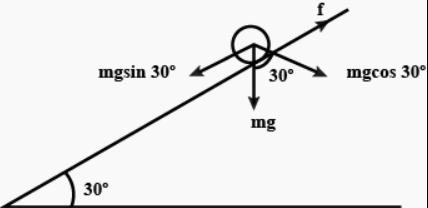The acceleration of the cylinder is given as: a = mg Sinθ / [m + (I/r2) ]
= mg Sinθ / [m + {(1/2)mr2/ r2} ]
= (2/3) g Sin 30°
= (2/3) × 9.8 × 0.5  =  3.27 ms-2

a. Using Newton’s second law of motion, we can write net force as:
fnet = ma
mg Sin 30° - f = ma
f = mg Sin 30° - ma
= 10 × 9.8 × 0.5 - 10 × 3.27
49 - 32.7 = 16.3 N

b. During rolling, the instantaneous point of contact with the plane comes to rest. Hence, the work done against frictional force is zero.

(c) For rolling without skid, we have the relation:
μ = (1/3) tan θ
tan θ = 3μ = 3 × 0.25
∴ θ = tan-1 (0.75) = 36.87°.

Question 32. Read each statement below carefully, and state, with reasons, if it is true or false;
a. During rolling, the force of friction acts in the same direction as the direction of motion of the CM of the body.
b. The instantaneous speed of the point of contact during rolling is zero.
c. The instantaneous acceleration of the point of contact during rolling is zero.
d. For perfect rolling motion, work done against friction is zero.
e. A wheel moving down a perfectly frictionless inclined plane will undergo slipping (not rolling) motion.

Solution :
a. False
Frictional force acts opposite to the direction of motion of the centre of mass of a body. In the case of rolling, the direction of motion of the centre of mass is backward. Hence, frictional force acts in the forward direction.
b. True
Rolling can be considered as the rotation of a body about an axis passing through the point of contact of the body with the ground. Hence, its instantaneous speed is zero.
c. False
This is becausehen a body is rolling, its instantaneous acceleration is not equal to zero. It has some value.
d. True
This is because once the perfect rolling begins, force of friction becomes zero. Hence work done against friction is zero.
e. True
This is because rolling occurs only on account of friction which is a tangential force capable of providing torque. When the inclined plane is perfectly smooth, the wheel will simply slip under the effect of its own weight.